HOW TWO SQ DECODERS CAN FUNCTION AS AN SQ ENCODER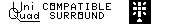Read the parent article to learn how to choose a mixer, and how to build and use the encoders.

Why two SQ decoders can function as an SQ encoder

Why two SQ decoders are needed:

Since the SQ decoders have only two inputs and four outputs, one SQ decoder is needed to encode the front, and one is needed for the back.

If sounds are intended to be panned across the front, or across the back, then only one SQ decoder is needed. The front panning does not require one.

But if the recording engineer wants to place sounds on the sides or the diagonal splits, then two SQ decoders are needed.

Actually, three SQ decoders are needed. One is needed to monitor the encoding.

The original 4−corners encoder:

The original SQ encoding equations can be obtained by doing all of the following:

1. Trade the left and right input lines (for the left back and right back signals).
2. Trade the left back and right back output lines (for the left and right encoded signals).
 The SQ 4−corners encoding equations are: l = LF − .7LBj + .7RB r = RF − .7LB + .7RBj The four SQ decoding equations are: lf = l rf = r lb = .7lj − .7r rb = .7l − .7rj The SQ front channels can be used as is. Just substitute the encoding signals: l = LF r = RF The SQ back channels are transposed as follows: Trade left for right at both the inputs and the outputs. rb = .7rj − .7l lb = .7r − .7lj Trade the equations: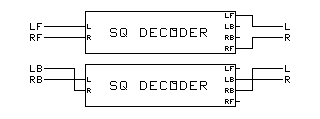lb = .7r − .7lj rb = .7rj − .7l Rearrange the terms: lb = − .7lj + .7r rb = − .7l + .7rj Now substitute the encoding signals: l = − .7LBj + .7RB r = − .7LB + .7RBj Mix together the outputs of the front and back encoders: l = LF − .7LBj + .7RB r = RF − .7LB + .7RBj Compare with the original encoder equations: l = LF − .7LBj + .7RB r = RF − .7LB + .7RBj Notice that they are the same.

The 4−corners and diagonal split encoder:

The original SQ encoding setup above can be modified by adding a phase inverter switch. Do all of the following:

1. Trade the left and right input lines (for the left back and right back signals).
2. Trade the left back and right back output lines (for the left and right encoded signals).
3. Add a switchable phase inverter to the left back input.

Features:

1. In the normal position, the encoder works 4−corners.
2. In the reversed phase position, it works for optimum diagonal splits.
 The SQ diagonal split encoding equations are: l = LF + .7LBj + .7RB r = RF + .7LB + .7RBj The four SQ decoding equations are: lf = l rf = r lb = .7lj − .7r rb = .7l − .7rj The SQ front channels can be used as is. Just substitute encoding signals: l = LF r = RF The SQ back channels are transposed as follows: Trade left for right at inputs and at outputs. rb = .7rj − .7l lb = .7r − .7lj Trade the equations: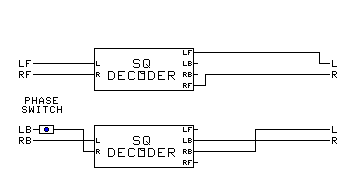lb = .7r − .7lj rb = .7rj − .7l Rearrange the terms: lb = − .7lj + .7r rb = − .7l + .7rj Now substitute the encoding signals: l = − .7LBj + .7RB r = − .7LB + .7RBj Reverse the phase of the left back input signal: l = .7LBj + .7RB r = .7LB + .7RBj Mix together the outputs of the front and back encoders: l = LF + .7LBj + .7RB r = RF + .7LB + .7RBj Compare with the original encoder equations: l = LF + .7LBj + .7RB r = RF + .7LB + .7RBj They are the same.

The 4−corners and forward−oriented encoder:

The original SQ encoding setup above can be modified by adding a different phase inverter switch. Do all of the following:

1. Trade the left and right input lines (for the left back and right back signals).
2. Add a switchable phase inverter and line trader to the back outputs.

Features:

• With the phase switch in the normal position, the encoder works 4−corners. The switch trades the left back and right back output lines.
• With the phase switch in the reversed phase position, it works for forward−oriented encoding. It reverses phase, but doesn't trade outputs.

The Normal−Interior switch can be added. In the interior position, it randomizes phase between front and back for acroperiphony.

 The SQ forward−oriented encoding equations are: l = LF + .7LB − .7RBj r = RF − .7LBj + .7RB The four SQ decoding equations are: lf = l rf = r lb = .7lj − .7r rb = .7l − .7rj The SQ front channels can be used as is. Just substitute encoding signals: l = LF r = RF The SQ back channels are transposed as follows: Trade left for right at inputs. lb = .7rj − .7l rb = .7r − .7lj Rearrange the terms:lb = − .7l + .7rj rb = − .7lj + .7r Now substitute the encoding signals: l = − .7LB + .7RBj r = − .7LBj + .7RB Reverse the phase of the left output signal: l = .7LB − .7RBj r = − .7LBj + .7RB Mix together the outputs of the front and back encoders: l = LF + .7LB − .7RBj r = RF − .7LBj + .7RB Compare with the original encoder equations: l = LF + .7LB − .7RBj r = RF − .7LBj + .7RB They are the same.The Triple Encoder: 4−corners, diagonal split, and forward−oriented encoder:

The original SQ encoding setup above can be modified by adding both phase inverter switches. Do all of the following:

1. Trade the left and right input lines (for the left back and right back signals).
2. Add a switchable phase inverter (1) to the left back input.
3. Add a switchable phase inverter and line trader (2) to the back outputs.
4. To add backward−oriented encoding too, add a switchable phase inverter (3) to the left front input.

The equations above apply.

Features:

• With phase switches 1 and 2 in the normal position, the encoder works 4−corners. Phase switch 2 trades left and right back output lines.
• With phase switch 1 reversed and switch 2 normal, the encoder works diagonal split. Switch 2 trades left and right back output lines.
• With phase switch 1 normal and switch 2 reversed, it works for forward−oriented encoding. Switch 2 reverses phase, but doesn't trade outputs.
• With phase switches 1, 2, and 3 reversed, it works for backward−oriented encoding.

The Normal−Interior switch can be added. In the interior position, it randomizes phase between front and back for acroperiphony.

The backward−oriented encoding equations are as follows.

 The SQ backward−oriented encoding equations are: l = − LF − .7LB − .7RBj r = RF + .7LBj + .7RB The four SQ decoding equations are: lf = l rf = r lb = .7lj − .7r rb = .7l − .7rj The SQ front channels get the left front phase inverted: l = − LF r = RF The SQ back channels are transposed as follows: Trade left for right at inputs. lb = .7rj − .7l rb = .7r − .7lj Rearrange the terms: lb = − .7l + .7rj rb = − .7lj + .7r Now substitute the encoding signals: l = − .7LB + .7RBj r = − .7LBj + .7RB Reverse the phase of the left output signal: l = .7LB − .7RBj r = − .7LBj + .7RB Reverse the phase of the left back input signal: l = − .7LB − .7RBj r = .7LBj + .7RB Mix together the outputs of the front and back encoders: l = − LF − .7LB − .7RBj r = RF + .7LBj + .7RB Compare with the original encoder equations: l = − LF − .7LB − .7RBj r = RF + .7LBj + .7RB They are the same.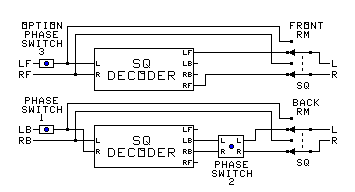The Triple Encoder in a switched RM−SQ setup:

The Triple encoding setup above can be modified by adding RM−SQ switches. Do all of the following:

1. Trade the left and right input lines (for the left back and right back signals).
2. Add a switchable phase inverter (1) to the left back input.
3. Add a switchable phase inverter and line trader (2) to the back outputs.
4. For backward−oriented encoding, add a switchable phase inverter (3) to the left front input.
5. Add the RM−SQ switches as shown.

The equations above apply.

Features:

• Using the SQ encoder
• With phase switches 1 and 2 in the normal position, the encoder works 4−corners. Phase switch 2 trades left and right back output lines.
• With phase switch 1 reversed and switch 2 normal, the encoder works diagonal split. Switch 2 trades left and right back output lines.
• With phase switch 1 normal and switch 2 reversed, it works for forward−oriented encoding. Switch 2 reverses phase, but doesn't trade outputs.
• With phase switches 1, 2, and 3 reversed, it works for backward−oriented encoding.
• Move the front switch to the RM position to get the SQ interior position for acroperiphony.
• Use the forward−oriented encoder as instructed here for basic UMX.
• Using the RM encoder
• With phase switch 1 in the normal position, the encoder encodes only the front half of the sound field. It can be used for stereo.
• With phase switch 1 reversed, the encoder works for normal RM.
• Switch 2 does nothing when encoding RM.
• Switch 3 in the reversed position changes front channel encoding into back channel encoding.
• Pan positions on the mixer control RM encoding, extending out into the sides.
• Move the front switch to the SQ position to get the RM interior position for acroperiphony.
• A different RM acroperiphony: front = SQ, Back = RM, Switch 1 = normal, Switch 3 = reverse. But the front and back input buses are traded.The Ultimate Encoder Pair (need at least two of these for full SQ):

The Switched encoding setup above can be modified by using a 3−position RM−SQF−SQB switch. Do all of the following:

1. Trade the left and right input lines (for the left back and right back signals).
2. Add a switchable phase inverter (1) to the left source (going to the right input).
3. Add a switchable phase inverter and line swap (2) to the back outputs.
4. Trade the left front and right front output lines.
5. Add the RM−SQF−SQB switch as shown.

The equations above apply.

Use table below to set the switches.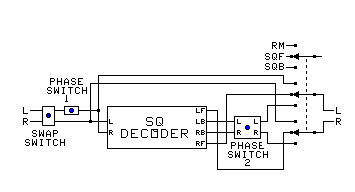The Ultimate Encoder Pair with CircleSurround capability:

The Ultimate Encoder Pair above can be modified for CircleSurround by adding the swap switch. Do all of the following:

1. Trade the left and right decoder input lines (for the left back and right back signals).
2. Put the swap switch at the mixer bus outputs.
3. Add a switchable phase inverter (1) to the left source (going to the right input).
4. Add a switchable phase inverter and line swap (2) to the back outputs.
5. Trade the left front and right front output lines.
6. Add the RM−SQF−SQB switch as shown.

The equations above apply.

Setting the switches:

SWITCH TABLE
OPERATION CHANNELS SWAP
SWITCH
PHASE
SWITCH
1
PHASE
SWITCH
2
MODE
SWITCH
PAN POT
CONTROLS
CONTROLS
Stereo Pass−Through Stereo Normal Normal RM L − F − R not used
Phased Normal Normal SQF L − F − R not used
RM Encoder Front Normal Normal RM L − LF − F − RF − R not used
Use Flip Buses
Back Normal Reverse RM L −LB − B − RB − R
RM Acroperiphonic Encoder Front Normal Normal RM L − LF − F − RF − R F − C − B
Back Normal Reverse SQF L − LB − B − RB − R
SQ 4−Corners Encoder Front Normal Normal SQF LF − F − RF not used
Use Flip Buses
Back Normal Normal Normal SQB LB − B − RB
SQ Diagonal−Split Encoder Front Normal Normal SQF LF − F − RF F − Split − B
Back Normal Reverse Normal SQB LB − F − RB
SQ Forward−Oriented Encoder Front Normal Normal SQF LF − F − RF F − Side − B
Back Normal Normal Reverse SQB LB − F − RB
SQ Backward−Oriented Encoder Front Normal Reverse SQF LF − B − RF F − Side − B
Back Normal Reverse Reverse SQB LB − B − RB
SQ Acroperiphonic Encoder Front Normal Normal RM LF − F − RF F − C − B
Back Normal Normal Normal SQB LB − B − RB
UMX Basic Encoder Left−Right Normal Normal SQF L − C − R [L−R] − C − [F−B]
Front−Back Normal Normal Reverse SQB F − C − B
RM 3D Front Encoder Front Normal Normal SQF L − LF − F − RF − R Horiz − Oblique − Vert
Down−Up Normal Normal Reverse SQB N − NF − F − ZF − Z
RM 3D Back Encoder Back Normal Reverse SQF L − LB − B − RB − R Horiz − Oblique − Vert
Down−Up Normal Reverse Reverse SQB N − NB − B − ZB − Z
CircleSurround Encoder Front Normal Normal SQF LF − F − RF F − Side − B
Back Swap Normal Reverse SQB LB − F − RB

Making an SQ Decoder behave as an encoder:

Disabling the 10−40 blend on a basic decoder:

Make the following changes:

1. Disconnect one end of the resistor connected between pin 2 and pin 11 of the MC1312P Integrated Circuit.
2. Disconnect one end of the resistor connected between pin 3 and pin 14 of the MC1312P Integrated Circuit.
3. If the decoder will be used for both a decoder and an encoder, add a double pole single throw to reconnect the resistors when desired.
4. If you do not want to modify the decoder, place a Phlazex unit on the outputs, and adjust it to cancel the blend.

Disabling the blend and logic on a Wavematching decoder:

Make the following changes:

1. Disconnect one end of the resistor connected between pin 2 and pin 6 of the MC1314P Integrated Circuit.
2. Disconnect one end of the resistor connected between pin 10 and pin 14 of the MC1312P Integrated Circuit.
3. If the decoder will be used for both a decoder and an encoder, add a double pole single throw to reconnect the resistors when desired.
4. Turn down the Dimension control.
5. If you do not want to modify the decoder, place a Phlazex unit on the outputs, and adjust it to cancel the blend.